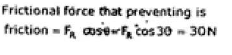Courses

# Engineering Mechanics

## 20 Questions MCQ Test GATE Mechanical (ME) 2022 Mock Test Series | Engineering Mechanics

Description
This mock test of Engineering Mechanics for GATE helps you for every GATE entrance exam. This contains 20 Multiple Choice Questions for GATE Engineering Mechanics (mcq) to study with solutions a complete question bank. The solved questions answers in this Engineering Mechanics quiz give you a good mix of easy questions and tough questions. GATE students definitely take this Engineering Mechanics exercise for a better result in the exam. You can find other Engineering Mechanics extra questions, long questions & short questions for GATE on EduRev as well by searching above.
QUESTION: 1

Solution:
QUESTION: 2

### The area moment of inertia about the centroidal axis of a rectangular section is given by 106667 mm4. If the width and depth of the section are 20mm and 40mm, then determine the moment of inertia about the base of the section.

Solution: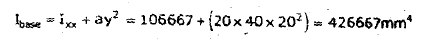QUESTION: 3

### A gear system is used to pull a load of 180 N as shown in figure (All dimensions are in cm). What will be the minimum force required to pull up the load?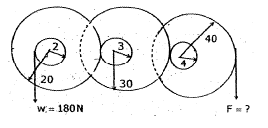Solution: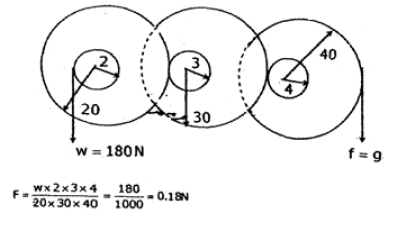QUESTION: 4

A block of mass 5 kg is placed on an inclined plane shows inclination is 30° to ground. Co-efficient of friction between surface and block is 0.3. Find out horizontal force on the block which is required to pull up the block on inclined plane.

Solution: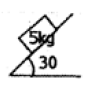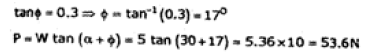QUESTION: 5

In a toggle joint shown in figure, find out horizontal force P. Vertical force at joint C is 5 N. Length of links are 3 m and 5 m.Solution: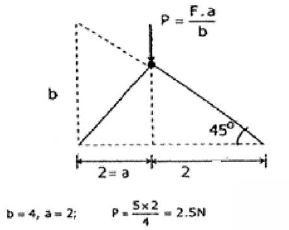QUESTION: 6

Fig. shows the two bodies of masses m1 and m2 connected by a light inextensible string and a passing over a smooth pulley. Mass m2 lies on a smooth horizontal plane.
When mass m1 is moving downward, the acceleration of the two bodies is equal to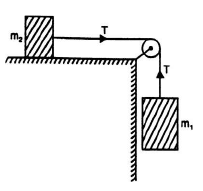Solution:
QUESTION: 7

The maximum height attained by a ball (of weight 500 N) which is thrown vertically upwards with a velocity 4.9 m/s is equal to

Solution:
*Answer can only contain numeric values
QUESTION: 8

A tower is of height 100 m. A stone is thrown up from the foot of water with a velocity of 20 m/s and at the same time another stone is dropped from the top of the tower. The two stones will meet after _________ s.

Solution:
QUESTION: 9

Two weights of 50 N and 150 N (of two blocks A and B respectively) are connected by a string and frictionless and weightless pulleys as shown in Fig. The tension in the string would be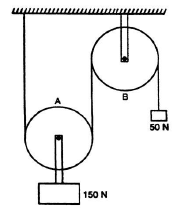Solution:
*Answer can only contain numeric values
QUESTION: 10

A ladder of weight 250 N is placed against a smooth vertical wall and a rough horizontal floor (µ = 0.3) as shown in Fig.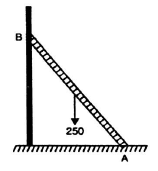If the ladder is on the point of sliding, the reaction at A will be __________ N.

Solution:
QUESTION: 11

A weight of 200 N is supported by two cables as shown in figure. The tension in the cable making angle θ will be minimum when the value of θ is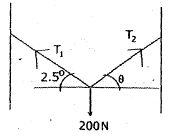Solution: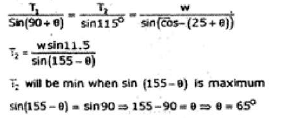QUESTION: 12

T​he three masses of 40 kg, 80 kg and 120 kg are supported as shown in figure. If masses are released simultaneously, then the tight cord will be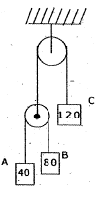Solution:
QUESTION: 13

A name board of 500 kg is supported by a horizontal bar and inclined cable at 60° as shown in figure. Neglect the weight of bar, tension in cable will be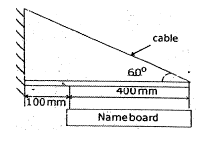Solution:
*Answer can only contain numeric values
QUESTION: 14

The load ‘W’ for the arrangement shown in figure will be ________ N.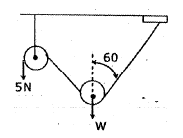Solution:
QUESTION: 15

In the given figure, find the angle at which the ladder loses its contact with the wall before it hits the ground? Assume centre of mass of ladder moves in a circle and friction less ground and wall.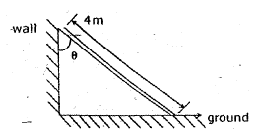Solution:
QUESTION: 16

Find out force ‘F’ required to pull the weight of 100 N using the pulley as shown in the figure.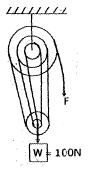Solution: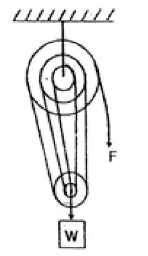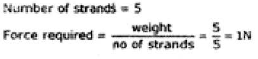QUESTION: 17

Common Data Questions 17-18

In a carnival ride, the passengers sit on a seat that rotates with constant speed in a vertical circle of radius 2 m. The heads of the seated passenger always pointed towards the axis of rotation and the carnival ride completes one full circle in 2 seconds.

Q. Find the acceleration of passenger

Solution: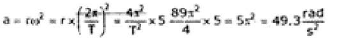QUESTION: 18

In a carnival ride, the passengers sit on a seat that rotates with constant speed in a vertical circle of radius 2 m. The heads of the seated passenger always pointed towards the axis of rotation and the carnival ride completes one full circle in 2 seconds.

Find the slowest rate of rotation if the seat belt is to exert no force on the passenger at the top of ride.

Solution: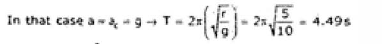QUESTION: 19

A ladder of mass 10 kg leans against a larger smooth sphere of radius 5 m which is fixed on a horizontal surface. The ladder makes an angle 60° with horizontal and having length 12.5 m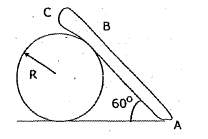What is the force that the sphere exerts on ladder?

Solution: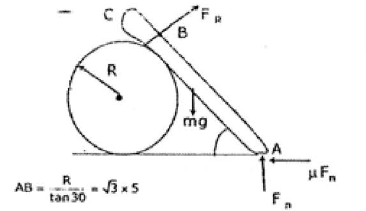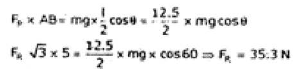QUESTION: 20

A ladder of mass 10 kg leans against a larger smooth sphere of radius 5 m which is fixed on a horizontal surface. The ladder makes an angle 60° with horizontal and having length 12.5 mWhat is frictional force preventing the ladder from slipping?

Solution: